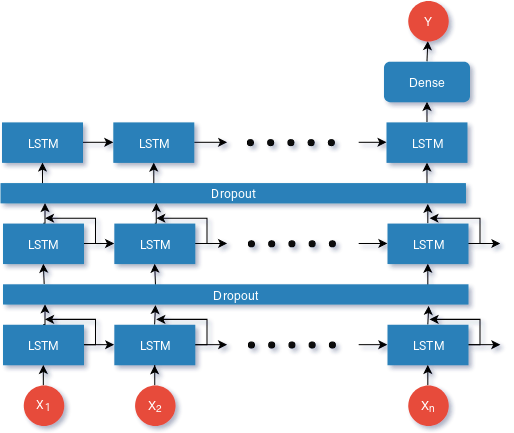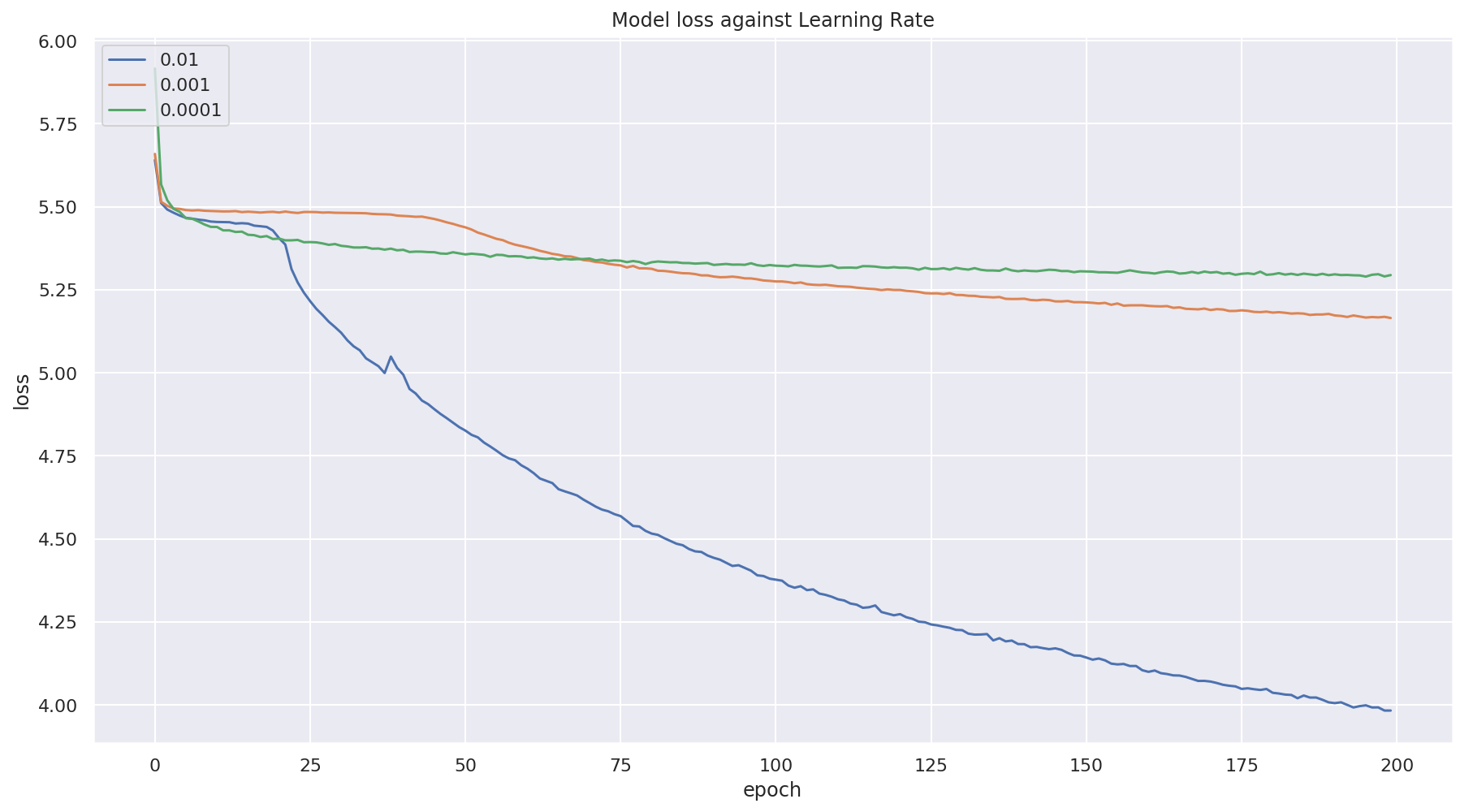Deeprock: LSTM based Rock Guitar Generator
Wed Jan 23, 2019 · 1184 words

Show me the code: https://github.com/KarthikNayak/DeepRock

I joined Recurse Center with a goal of learning more about deep-learning. One of the projects I wanted to work on was:

Given a sequence of musical notes (X), can you train a Deep Neural Network to learn improvisation over those notes and come up with its own music (Y).

This post is a step in that direction. First we shall take it one level easier and try to train a model which can learn the intricacies of a Lead guitar and is able to Generate its own composition from the training received.

## Obtaining the data

We shall use this dataset of midi files as our source for this training.

#### What is MIDI?

MIDI is short for Musical Instrument Digital Interface. Lets think of it as a way to store instrument data, wherein we can think of each song as something which contains one or more instruments (AKA tracks). Each track is said to contain one or more musical notes. Each note is an aggregation of:

1. Pitch of the note
2. Volume of the note
3. Time step relative to previous note

MIDI also contains a lot more data and also metadata related to the said data. But we don’t really care about all that for now.

#### Parsing the data

An important step of all Deep learning projects is ensuring that we parse the data and have good clean data for the model to learn on.

So our plan here is to:

1. Filter out only those MIDI files which have a ‘Guitar Instrument’ in them.
2. Get all notes form the filtered MIDI tracks for the ‘Guitar Instrument’.
3. Split these notes into batches of length `sequence_length + 1`.
4. Only pick sequences which have a minimum of `unique_factor` number of unique notes in them.
5. Now our `X` will be `data[0:sequence_length]` and `Y` will be `data[sequence_length]`
6. One hot encode `Y`. Normalize and Standardize `X`.

Code for the same:

``````# Parse the MIDIs to get only tracks with Guitars in them
def populate_guitar_track():
guitar_parts = []
for file in glob.glob("midi/**/*.mid", recursive=True):
try:
score = converter.parse(file)
guitar = instrument.ElectricGuitar
for part in instrument.partitionByInstrument(score):
if isinstance(part.getInstrument(), guitar):
print(f"Has Guitar: {file}")
guitar_parts.append(file)
except:
continue

# Generator to go through Guitar MIDIs and yield the tracks
def get_tracks():
with open('object/data/guitar_midi_files', 'rb') as f:
for file in guitar_parts:
print(f"In file: {file}")
song = converter.parse(file)
for part in instrument.partitionByInstrument(song):
if isinstance(part.getInstrument(), instrument.ElectricGuitar):
yield part

# Get all notes from a given track
def get_notes(seq_len=1, reset=False):
data = []
print(f"Parsing notes with reset set to {reset}")
if not reset:
with open('object/data/notes', 'rb') as f:
print(f"Returning notes from pickle")
for track in get_tracks():
tmp = []
notes = track.recurse()
for n in notes:
if isinstance(n, note.Note):
tmp.append(str(n.pitch))
elif isinstance(n, chord.Chord):
tmp.append(' '.join(str(x.pitch) for x in n))
tmp = tmp[:int(len(tmp)/seq_len)*seq_len]
data.extend(tmp)
print(f"Done parsing notes")
return data

# Check if the number of unique notes confirms to our minimum requirement
def check_data(data):
if len(np.unique(data)) > unique_factor:
return True
return False

# Parse notes and creating Training data
def create_training_data(reset=False):
X = []
Y = []
data = get_notes(sequence_length, reset)
idx = int(len(data) * data_percent/100)

enc = OrdinalEncoder()
enc.fit(np.array(data).reshape(-1, 1))

print(f"Creating data from notes of size: {len(data)}")

for i in range(0, idx - sequence_length):
if check_data(data[i:i+sequence_length]):
X.append(enc.transform(np.reshape(
data[i:i+sequence_length], (-1, 1))))
Y.append(enc.transform(np.reshape(
data[i+sequence_length], (-1, 1))))

X = np.array(X)
Y = np.array(Y)

mean = X.mean()
std = X.std()
X = (X - mean) / std

onehot = OneHotEncoder(sparse=False)
Y = onehot.fit_transform(Y.reshape(-1, 1))``````

## Model ArchitectureThis the model we’ll be using. Some notes here:

1. We’ll be using the `Adam` optimizer
2. Loss will be calculated with `Categorical Cross Entropy`. This is because our `Y` values are One-hot Encoded.
3. We will iterate this training over `learning rates` of 0.01, 0.001 and 0.0001.
4. We run 200 `epochs` for each given learning rate.

Code for the same:

``````def create_network(input_shape, op_len, lr):
model = Sequential()

opt = keras.optimizers.Adam(lr=lr, beta_1=0.9, beta_2=0.999, decay=0.01)
model.compile(loss='categorical_crossentropy',
optimizer=opt, metrics=['accuracy'])
return model

def train():
x, y = get_xy()
onehot = None
with open('object/data/onehot', 'rb') as file:

for lr in [0.01, 0.001, 0.0001]:
print(f"Training with lr: {lr}")
model = create_network(x.shape[1:], onehot.categories_.shape, lr)
history = model.fit(x, y, epochs=200, batch_size=64)``````

## Results

The loss against various learning rates are shown below### Music Generation

Once the model is trained, generating it is simple.

1. We take notes of `sequence_length` as seed.
2. We use the seed notes for generating `Y`.
3. We now set the new seed as `prevNotes[1:sequence_length] + Y`.
4. Repeat

Enough talk, wheres the music at?

Music 1
Music 2
Music 3

Note: Music generated above contains the seed given to the generator too.

## Key Takeaways

#### Data Cleanup and Parsing

When I started off with this project, my expectation was to finish the project within 1 week. I undershot by a mile. It took exactly two weeks to actually wrap it up. This is mostly because I didn’t expect so much clean up would be required for the data I had gotten.

I’ve come to realize that with any Deep learning project, A good amount of time is spent in the data collection and aggregation stage. This is due to one of the following reasons

1. Data tends to be inconsistent in many forms. Here although the data set was huge, most of it was junk. This is because the dataset was mostly a collection of user submitted MIDIs which didn’t adhere to any quality control.
2. Data can be missing. Some MIDI files, didn’t contain metadata of which track belonged to which instrument. I even tried writing some logic to parse these tracks and do a probabilistic guess as to which track could be for the guitar. Although this worked on my small test set. I couldn’t scale if efficiently
3. Data can be repeated. Although this is minimal, I’ve noticed that parts of the data is repeated. This again is of two types WRT our context
• Multiple versions of a songs MIDI. This is because multiple users have submitted a MIDI for the same file. This means we have repeated information for some songs. We’ve ignored this constraint.
• Songs can have repeated notes. This is especially true in Rock music, where the guitar could repeat a sequence of notes over the song. We try to eliminate this using the `unique_factor` variable.

#### Hyper-Parameter Modification

I always overlooked this whenever I’ve gone through tutorials for deep-learning. But I cannot stress on how important this is. Hyper-Parameter tuning is really really important.

Having the right Hyper-Parameters can be deciding factor between a successful model and a model that doesn’t work at all.

I was stuck for a day with a model which was predicting the same output irrespective of the data being fed to the model. I couldn’t figure out why this was happening, as a last resort I tried to change the learning rate. This simple change got me a working model. Surprising.